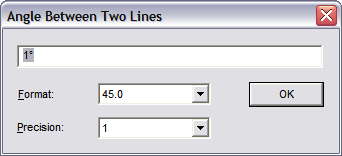# Angle between Two Lines

Measures the angle between two non-parallel lines and displays the result.

Point 1: The first line of the angle to be measured

NOTE: For a 3D solid, the Volume command calculates both surface area and volume.

Point 2: The second line of the angle to be measured

The result is displayed in the window.Format: Determine how you want the angle displayed.

Precision: Controls how many digits appear after the decimal point.